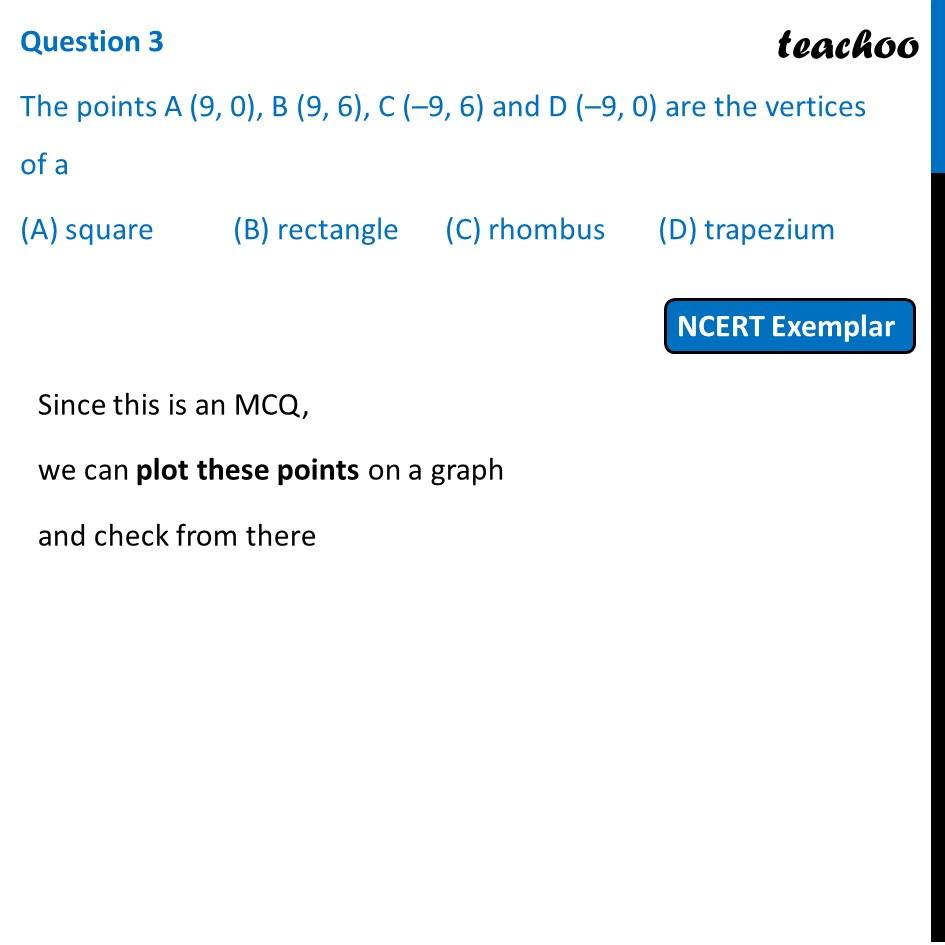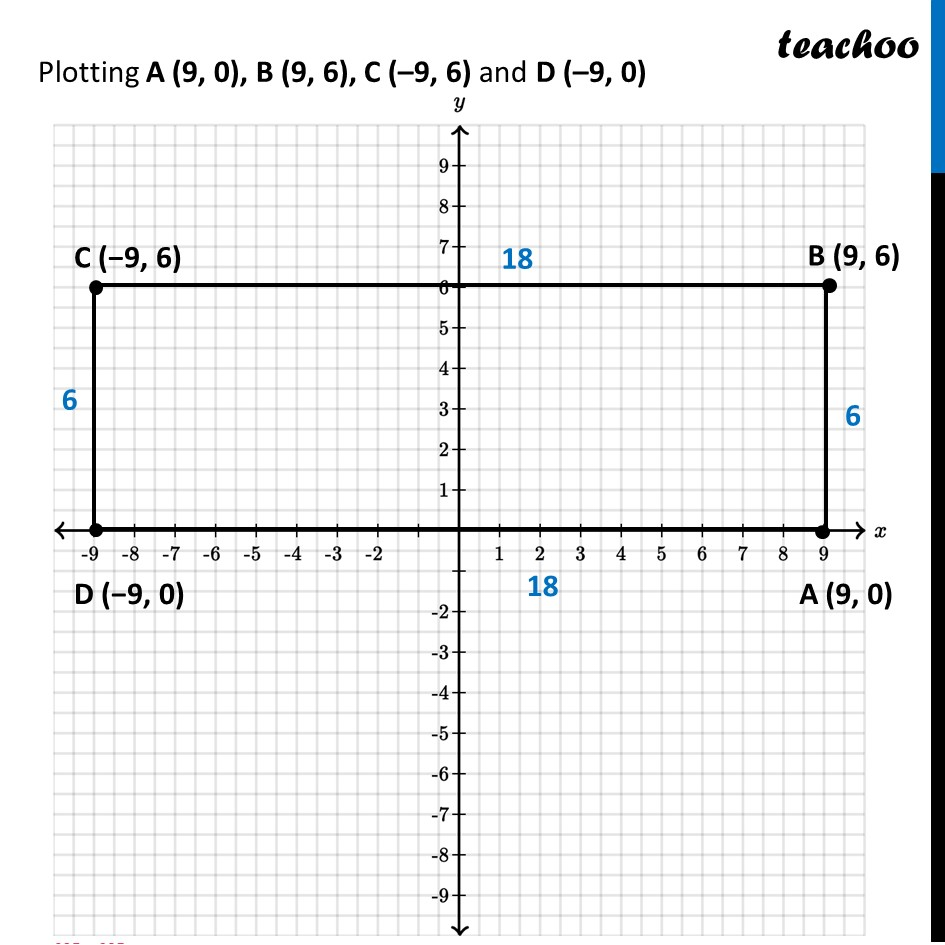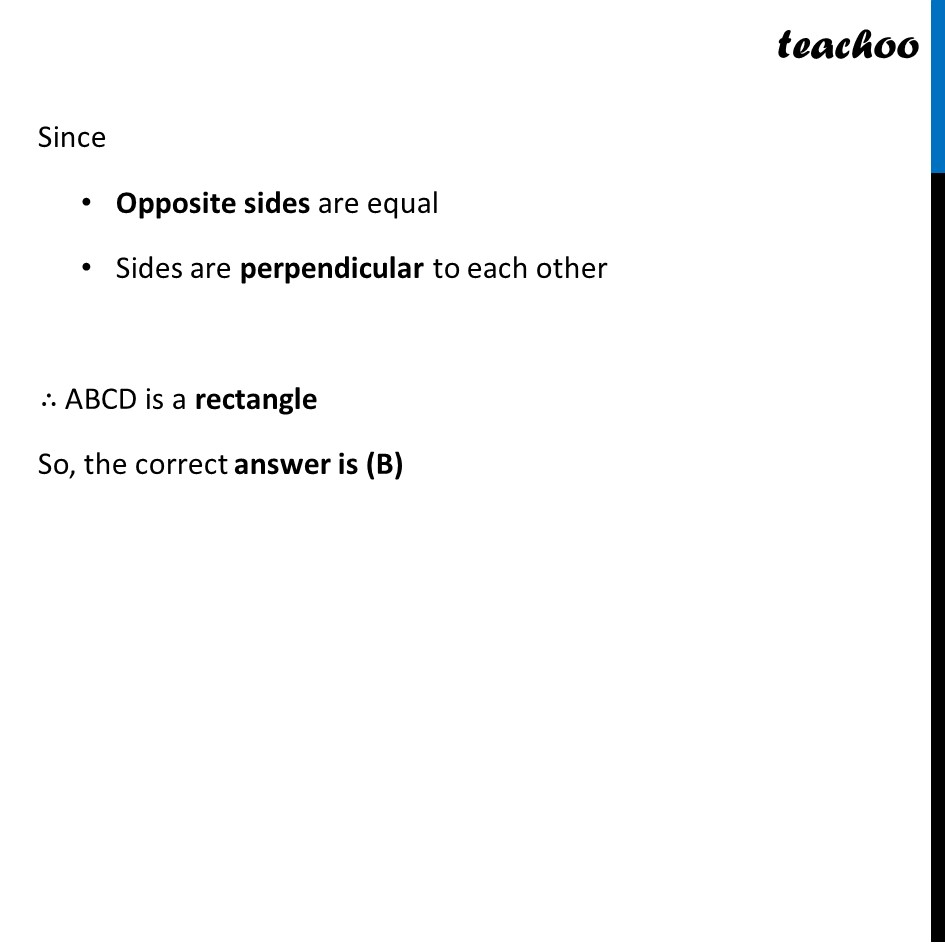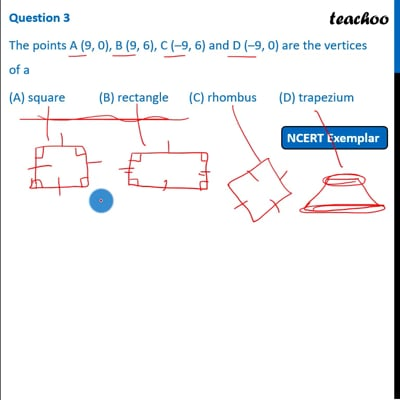NCERT Exemplar - MCQ

Chapter 7 Class 10 Coordinate Geometry
Serial order wise

## (A) square   (B) rectangle   (C) rhombus   (D) trapeziumThis video is only available for Teachoo black users

Maths Crash Course - Live lectures + all videos + Real time Doubt solving!

### Transcript

Question 3 The points A (9, 0), B (9, 6), C (–9, 6) and D (–9, 0) are the vertices of a (A) square (B) rectangle (C) rhombus (D) trapezium Since this is an MCQ, we can plot these points on a graph and check from there Plotting A (9, 0), B (9, 6), C (–9, 6) and D (–9, 0) Since Opposite sides are equal Sides are perpendicular to each other ∴ ABCD is a rectangle So, the correct answer is (B)